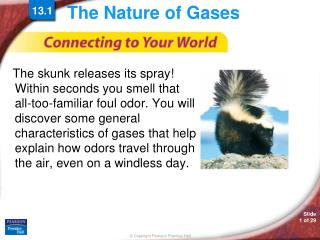Download PresentationThe Nature of Gases

# The Nature of Gases - PowerPoint PPT PresentationDownload Presentation## The Nature of Gases

- - - - - - - - - - - - - - - - - - - - - - - - - - - E N D - - - - - - - - - - - - - - - - - - - - - - - - - - -
##### Presentation Transcript

1. The Nature of Gases 13.1 • The skunk releases its spray! Within seconds you smell that all-too-familiar foul odor. You will discover some general characteristics of gases that help explain how odors travel through the air, even on a windless day.

2. 13.1 Kinetic Theory and a Model for Gases • Kinetic Theory and a Model for Gases • What are the three assumptions of the kinetic theory as it applies to gases?

3. 13.1 Kinetic Theory and a Model for Gases • The word kinetic refers to motion. • The energy an object has because of its motion is called kinetic energy. • KE = ½ *m *v2 • Temperature is a measure of the average kinetic energy of a substance. • According to the kinetic theory, all matter consists of tiny particles that are in constant motion.

4. 13.1 Kinetic Energy and Temperature • Kinetic Energy and Temperature • What is the relationship between the temperature in kelvins and the average kinetic energy of particles?

5. 13.1 Kinetic Energy and Temperature • Average Kinetic Energy • The particles in any collection of atoms or molecules at a given temperature have a wide range of kinetic energies. Most of the particles have kinetic energies somewhere in the middle of this range.

6. 13.1 Kinetic Energy and Temperature

7. 13.1 Kinetic Energy and Temperature • Absolute zero (0 K, or –273.15°C) is the temperature at which the motion of particles theoretically ceases. • Particles would have no kinetic energy at absolute zero. • Absolute zero has never been produced in the laboratory.

8. 13.1 Kinetic Energy and Temperature • Average Kinetic Energy and Kelvin Temperature • The Kelvin temperature of a substance is directly proportional to the average kinetic energy of the particles of the substance.

9. 13.1 Kinetic Energy and Temperature • In this vacuum chamber, scientists cooled sodium vapor to nearly absolute zero.

10. 13.1 Kinetic Theory and a Model for Gases • According to kinetic theory for an IDEAL GAS: • The particles in a gas are considered to be small, hard spheres with an insignificant volume. • The motion of the particles in a gas is rapid, constant, and random. • All collisions between particles in a gas are perfectly elastic.

11. 13.1 Kinetic Theory and a Model for Gases • Particles in a gas are in rapid, constant motion.

12. 13.1 Kinetic Theory and a Model for Gases • Gas particles travel in straight-line paths.

13. 13.1 Kinetic Theory and a Model for Gases • The gas fills the container.

14. Compressibility • Compressibility is a measure of how much the volume of matter decreases under pressure. When a person collides with an inflated airbag, the compression of the gas absorbs the energy of the impact.

15. Low Denisty • At room temperature, the distance between particles in an enclosed gas is about 10 times the diameter of a particle.

16. 13.1 Gas Pressure • Gas Pressure • How does kinetic theory explain gas pressure?

17. 13.1 Gas Pressure • Gas pressure results from the force exerted by a gas per unit surface area of an object. • An empty space with no particles and no pressure is called a vacuum. • Atmospheric pressure results from the collisions of atoms and molecules in air with objects.

18. 13.1 Gas Pressure • Gas pressure is the result of simultaneous collisions of billions of rapidly moving particles in a gas with an object.

19. 13.1 Gas Pressure • A barometer is a device that is used to measure atmospheric pressure.

20. 13.1 Gas Pressure • The SI unit of pressure is the pascal (Pa). • One standard atmosphere (atm) is the pressure required to support 760 mm of mercury in a mercury barometer at 25°C.

21. 13.1

22. 13.1

23. 13.1

24. 13.1

25. for Sample Problem 13.1

26. Kinetic Energy and Temperature • Animation 14 • Observe particles in motion and discover the connection between temperature and kinetic energy.

27. 13.1 Section Quiz. • 1. According to the kinetic theory, the particles in a gas • are attracted to each other. • are in constant random motion. • have the same kinetic energy. • have a significant volume.

28. 13.1 Section Quiz. • 2. The pressure a gas exerts on another object is caused by • the physical size of the gas particles. • collisions between gas particles and the object. • collisions between gas particles. • the chemical composition of the gas.

29. 13.1 Section Quiz. • 3. The average kinetic energy of the particles in a substance is directly proportional to the • Fahrenheit temperature. • Kelvin temperature. • molar mass of the substance. • Celsius temperature.